# Pulse-Shape Filtering in Communications Systems

## Overview

This tutorial is part of the National Instruments Measurement Fundamentals series. Each tutorial in this series, will teach you a specific topic of common measurement applications, by explaining the theory and giving practical examples. This tutorial covers an introduction to RF, wireless and high-frequency signals and systems.

For the complete list of tutorials, return to the NI Measurement Fundamentals Main page or for more RF tutorials refer to the NI RF Fundamentals main subpage. For more information on National Instruments RF products, visit www.ni.com/rf.

In a digital communication system, digital information can be sent on a carrier through changes in its fundamental characteristics such as: phase, frequency, and amplitude. In a physical channel, these transitions can be smoothed, depending on the filters implemented in transmission. In fact, the use of a filter plays an important part in a communications channel because it is effective at eliminating spectral leakage, reducing channel width, and eliminating interference from adjacent symbols (Inter Symbol Interference, ISI).

## Pulse Shaping Fundamentals

In communications systems, two important requirements of a wireless communications channel demand the use of a pulse shaping filter. These requirements are: 1) generating bandlimited channels, and 2) reducing inter symbol interference (ISI) from multi-path signal reflections. Both requirements can be accomplished by a pulse shaping filter which is applied to each symbol. In fact, the sinc pulse, shown below, meets both of these requirements because it efficiently utilizes the frequency domain to utilize a smaller portion of the frequency domain, and because of the windowing affect that it has on each symbol period of a modulated signal. A sinc pulse is shown below along with an FFT spectrum of the given signal.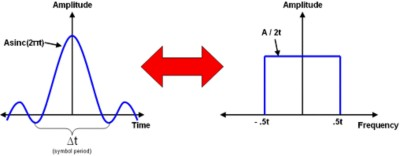Figure 1: Time vs. Frequency Domain for a Sinc Pulse

As the figure illustrates, the sinc pulse is periodic in nature and it has a maximum amplitude in the middle of the symbol time. In addition, it appears as a square wave in the frequency domain and thus can effectively limit a communications channel to a specific frequency range.

## Reducing Channel Bandwidth

Fundamentally, modulation of a carrier sinusoid results in constant transitions in its phase and amplitude. Below, we show the time domain of a carrier sinusoid with a symbol rate that is half of the carrier. We know this relationship because the phase/amplitude transitions occur at every two periods of the carrier. As you can see from the diagram, sharp transitions occur when filtering is not applied.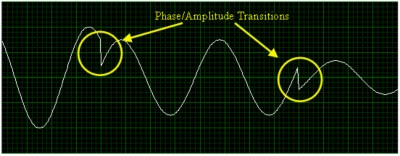Figure 2: Phase and Amplitude Transitions in an Unfiltered Modulated Signal

As one might guess, sharp transitions in any signal result in high-frequency components in the frequency domain. In the graph at right, we show an FFT of the unfiltered signal. As expected, the graph illustrates significant channel power outside of the 1 GHz bandwidth that we would expect. In a multi-channel communications systems, limiting all the power of a modulated carrier to just the carrier bandwidth is extremely important for several reasons. First, the transmission power is reduced when the signal has a more concentrated frequency range. In addition, limiting a channel to a specific frequency band eliminates adjacent channel interference.

By applying a pulse-shaping filter to the modulated sinusoid, the sharp transitions are smoothed and the resulting signal is limited to a specific frequency band. Below, we show the time-domain modulated sinusoid.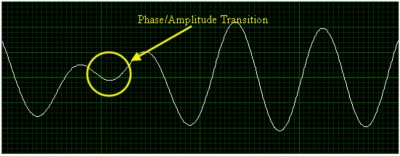Figure 3: Smoothed Phase and Amplitude Transitions in a Filtered Modulated Signal

As this image illustrates, the phase and amplitude transitions happen much more gradually when filtering is implemented. As a result, the frequency information of the sinusoid becomes more concentrated into a specified frequency band. We are able to see this by taking an FFT of the signal.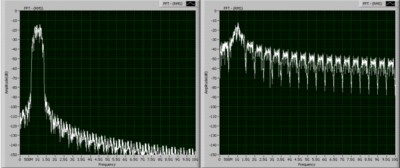Figure 4: Frequency Domain of Filtered vs. Unfiltered Signal

As you might expect, the sharp transitions do cause high frequency components in the frequency domain. Now, once a filter has been applied to the carrier signal, these high frequency components of the signal have been removed. Thus, the majority of the channel power is now limited to a specific defined bandwidth. Note that the required bandwidth for a channel is directly related to the symbol rate and is centered at the carrier frequency.

## Reducing Inter-Symbol Interference (ISI)

In bandlimited channels, intersymbol interference (ISI) can be caused by multi-path fading as signals are transmitted over long distances and through various mediums. More specifically, this characteristic of the physical environment causes some symbols to be spread beyond their given time interval. As a result, they can interfere with the following or preceding transmitted symbols. One solution to this problem is the application of the pulse shaping filter that we have previously described. By applying this filter to each symbol that is generated, we are able to reduce channel bandwidth while reducing ISI.
In addition, it is common to apply a match filter on the receiver side to minimize these affects. Below, we illustrate the implementation of a pulse shaping filter on each symbol that is generated. As the image illustrates, the maximum amplitude of the pulse-shaping filter occurs in the middle of the symbol period. In addition, the beginning and ending portions of the symbol period are attenuated. Thus, ISI is reduced by providing a pseudo-guard interval which attenuates signals from multi-path reflections.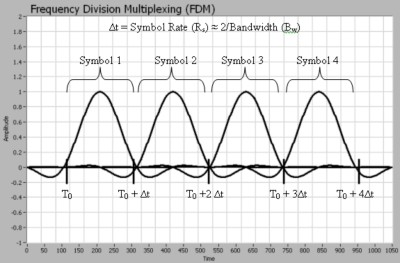Figure 5: Filter Implementation in the Time Domain

As we can see from the illustration above, the sinc pulses from subsequent symbols actually overlap on one another. However, because the peak of each sinc pulse corresponds with the zero crossing point of the following sinc pulse, intersymbol interference (ISI) is minimized.

## Matched Filtering

The matched filter is perhaps equally as important as the pulse-shaping filter. While the pulse shaping filter serves the purpose of generating signals such that each symbol period does not overlap, the matched filter is important to filter out what signal reflections do occur in the transmission process. Because a direct-path signal arrives at the receiver before a reflected signal does, it is possible for the reflected signal to overlap with a subsequent symbol period. This is illustrated in the diagram below: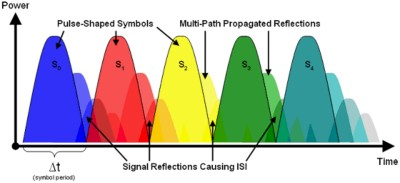Figure 6: ISI Caused by Multi-Path Distortion

As you can see, the matched filter reduces this affect by attenuating the beginning and ending of each symbol period. Thus, it is able to reduce intersymbol interference. One of the most common choices for a matched filter is the root raised cosine filter described above.

## Pulse Shaping in the NI LabVIEW Modulation Toolkit

In LabVIEW’s modulation toolkit, several different types of sinc pulses can be applied to the modulated signal to implement a pulse-shaping filter. These are the raised cosine filter, the root raised cosine filter, and the Gaussian filter. Each of these is discussed below.

Raised Cosine Filter
The raised cosine filter is one of the most common pulse-shaping filters in communications systems. In addition, it is used to minimize intersymbol interference (ISI) by attenuating the starting and ending portions of the symbol period. Because these portions are most susceptible to creating interference from multi-path distortion, the shaping characteristics of the raised cosine filter helps reduce ISI. This impulse response for this filter is given by the equation shown below: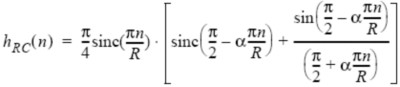As the equation shows, the sinc pulse is implemented in the creation of this filter. The filter rolloff parameter, alpha(α), can range between values of 0 and 1. The impulse response of the resulting filter is shown below: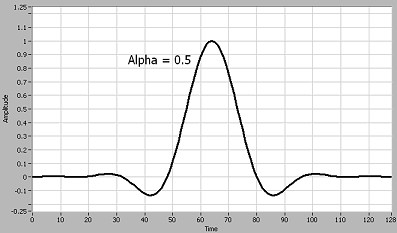Figure 7: Impulse Response of Raised Cosine Filter

Again, the sinc pulse is shaped such that ideally, the resulting channel bandwidth will be described by the equation:
Bw = Rs (1 + α)
Ideally, the frequency response (FFT) of the sinc pulse should yield a completely square response such that a specific frequency bandwidth (Bw is exactly half of the symbol rate (Rs). However, in the non-ideal programming environment, the actual frequency response differs slightly from the ideal response (due to estimation of infinity, ∞). Below, we show the non-ideal frequency response simply by taking the FFT (logarithmic) of the impulse response. This is shown below: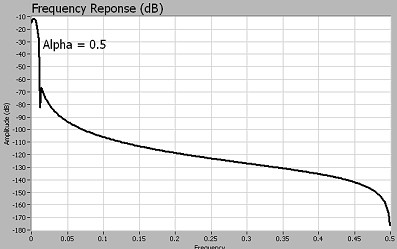Figure 8: Frequency Response of Raised Cosine Filter

In this specific example, we’ve used a rolloff factor (α) of 0.5. Note that the bandwidth of the signal is concentrated in a specific frequency range. Again this is critical in a communications system because keeping channels bandlimited is necessary to prevent adjacent channel interference.

Root Raised Cosine Filter
The root raised cosine filter produces a frequency response with unity gain at low frequencies and complete at higher frequencies. It is commonly used in communications systems in pairs, where the transmitter first applies a root raised cosine filter, and then the receiver then applies a matched filter.
Mathematically, the raised cosine filter can be defined by the following equation: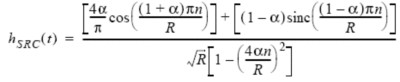In this equation, α is the rolloff factor, which determines the sharpness of the frequency response. In addition, R is the number of samples per symbol. As the equation above illustrates, the sinc pulse is used to shape the filter so that it appears with a finite frequency response. The impulse response for this filter is shown below: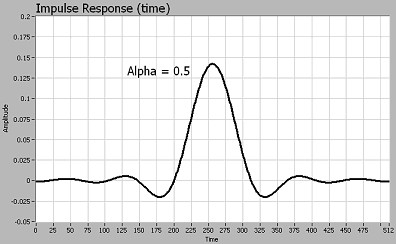Figure 9: Impulse Response of Root Raised Cosine Filter

As the graph illustrates, the impulse response resembles the sinc pulse described previously. As mentioned earlier, this should ideally have a perfectly square frequency response. We show the actual frequency response of the root raised cosine filter below: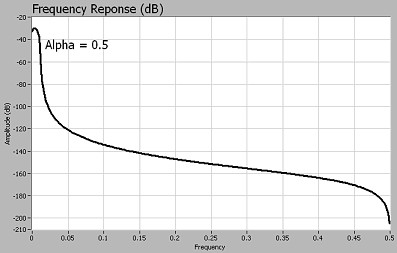Figure 10: Frequency Response of Root Raised Cosine Filter

Again, note from the figure above that an FFT of the impulse response is not completely ideal due to estimation of infinity. Again, we’ve used a rolloff factor (α) of 0.5. Also note that like the raised cosine filter, the bandwidth of the signal is concentrated in a specific frequency range.

Gaussian Filter
The Gaussian filter is a pulse shaping technique that is typically used for frequency shift keying (FSK) and minimum shift keying (MSK) modulation. This filter is unlike the raised cosine and root raised cosine filters because it does not implement zero crossing points. The impulse response for the Gaussian filter is defined by the following equation: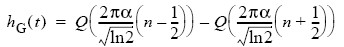Below, we show a graphical representation of the impulse response. As described above, note that there are no zero crossings for this type of filter.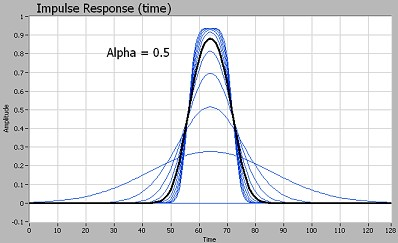Figure 11: Impulse Response of Gaussian Filter

## Filtering in Communications Systems

Fundamentally, many digital communication protocols are designed so that each channel is assigned to a specific frequency range. As we have already discussed, this can be implemented through the use of a pulse shaping filter. Note that the pulse shaping filter not only reduces intersymbol interference (ISI), but that it also reduces adjacent channel interference. Thus, pulse shaped filtering allows for the implementation of frequency division multiplexing (FDM) and a subset of FDM known as orthogonal frequency division multiplexing (OFDM).

## Frequency Divison Multiplexing

With frequency division multiplexing (FDM), each channel being assigned to a unique frequency range. Frequency division multiplexing is used in a variety of communications protocols. Some of the most common are data protocols such as Bluetooth and cellular protocols such as Bluetooth, GSM, TDMA, and CDMA. Again, each of these divides their allotted frequency bandwidth into smaller channels to allow multiple devices to communicate at the same time.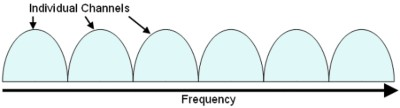Figure 12: Bandwidth Allocations for an FDM Communications System

Bluetooth, for example, is a digital communications protocol that is utilized by cell phones, laptops, and PDA’s. Bluetooth operates in the 2.4GHz unlicensed band and implements FDM by defining 79 channels from 2.402 GHz to 2.480 GHz which are spaced at 1 MHz apart. Each channel is bandlimited through the implementation of a Gaussian filter. The Bluetooth protocol specifically implements a Gaussian filter with an alpha (α) of 0.5.

As second common implementation of FDM is in the Global System for Mobile Communications protocol; which is better known as GSM. With GSM, the all communication channels are divided into 124 uplink and 124 downlink channels. The downlink channels fall within the 890 - 915 MHz range and the uplink channels are 935 - 960 MHz. Each channel is spaced by 200 kHZ. This protocol specifically uses a Gaussian filter (alpha = 0.3).

## Orthogonal Frequency Division Multiplexing

OFDM is a subset of frequency division multiplexing in which a single channel bandwidth utilizes multiple sub-carriers on adjacent frequencies. In addition the sub-carrier in an OFDM system is overlapping to maximize spectral efficiency. Ordinarily, overlapping adjacent channels can interfere with one another. However, in an OFDM system, each sub-carrier is orthogonal to one another. The significance of overlapping orthogonal channels is that they can overlap without interfering with one another. Thus, spectral efficiency is maximized without causing adjacent channel interference. This is represented in the diagram below.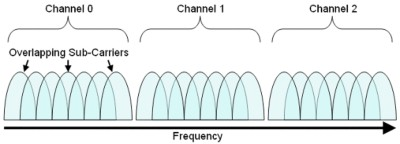Figure 13: Bandwidth Allocations for an OFDM Communications System

Notice above that each of the sub-carriers overlap with one another and that multiple sub-carriers are utilized for each individual channel. As a result of using overlapping sub-carriers for each channel, OFDM is able to maximize spectral efficiency. In addition, it is also resilient to multi-path channel interference.

Several common commercial protocols, such as digital video broadcast (DVB), asymmetric digital subscriber line (ADSL), and wireless Ethernet (WiFi) implement OFDM. More specifically the flavors of WiFI that implement OFDM are IEEE 802.11a and IEEE 802.11g. With these implementations, each channel occupies 16.25 MHz of bandwidth at the 2.4GHz frequency range. In addition, each channel is divided into 52 sub-carriers of 312.5 kHz. Together, these subcarriers overlap to fully utilize the 16.25 MHz channel bandwidth dedicated per channel. In addition, each sub-carrier can use BPSK, QPSK, 16-QAM, or 64-QAM modulation to optimize that data rate for a given physical channel.

## Conclusions and References

As we have seen, filters are an important part of communications systems. Above, we have discussed how they are used to eliminate spectral leakage, reduce channel width, and eliminate interference from adjacent symbols (Inter Symbol Interference, ISI). In LabVIEW, the modulation toolkit is able to be a fundamental building block of communications systems through the implementations of several types of filters.

For the complete list of tutorials, return to the NI Measurement Fundamentals Main page or for more RF tutorials refer to the NI RF Fundamentals main subpage. For more information on National Instruments RF products, visit www.ni.com/rf.

1. C. Richard Johnson and William A. Sethares, Telecommunications Breakdown.
2. John G. Proakis and Masoud Salehi, Communications Systems Engineering.
3. Simon Haykin, Communications Systems.
4. B.P. Lathi, Modern Digital and analog Communications Systems.
5. John G. Proakis, Digital Communications.
6. Theodore S. Rappaport, Wireless Communications: Principles and Practice.

## Related Products

NI PXI-5660 2.7 GHz RF Vector Signal Analyzer
The National Instruments PXI-5660 is a modular 2.7 GHz RF vector signal analyzer with 20 MHz of real-time bandwidth optimized for automated test.

NI PXI-5671 2.7 GHz RF Vector Signal Generator

The National Instruments PXI-5671 module is a 3-slot RF vector signal generator that delivers signal generation from 250 kHz to 2.7 GHz, 20 MHz of real-time bandwidth and up to 512 MB of memory.

NI PXI-5652 6.6 GHz RF and Microwave Signal Generator

The National Instruments PXI-5652 6.6 GHz RF and microwave signal generator is continuous-wave with modulation capability. It is excellent for setting up stimulus response applications with RF signal analyzers.

NI RF Switches

The National Instruments RF switch modules are ideal for expanding the channel count or increasing the flexibility of systems with signal bandwidths greater than 10 MHz to bandwidths as high as 26.5 GHz.

NI LabVIEW

National Instruments LabVIEW is an industry-leading graphical software tool for designing test, measurement, and automation systems.